BROWSE BY

#### Author

Results 1 - 7 of 7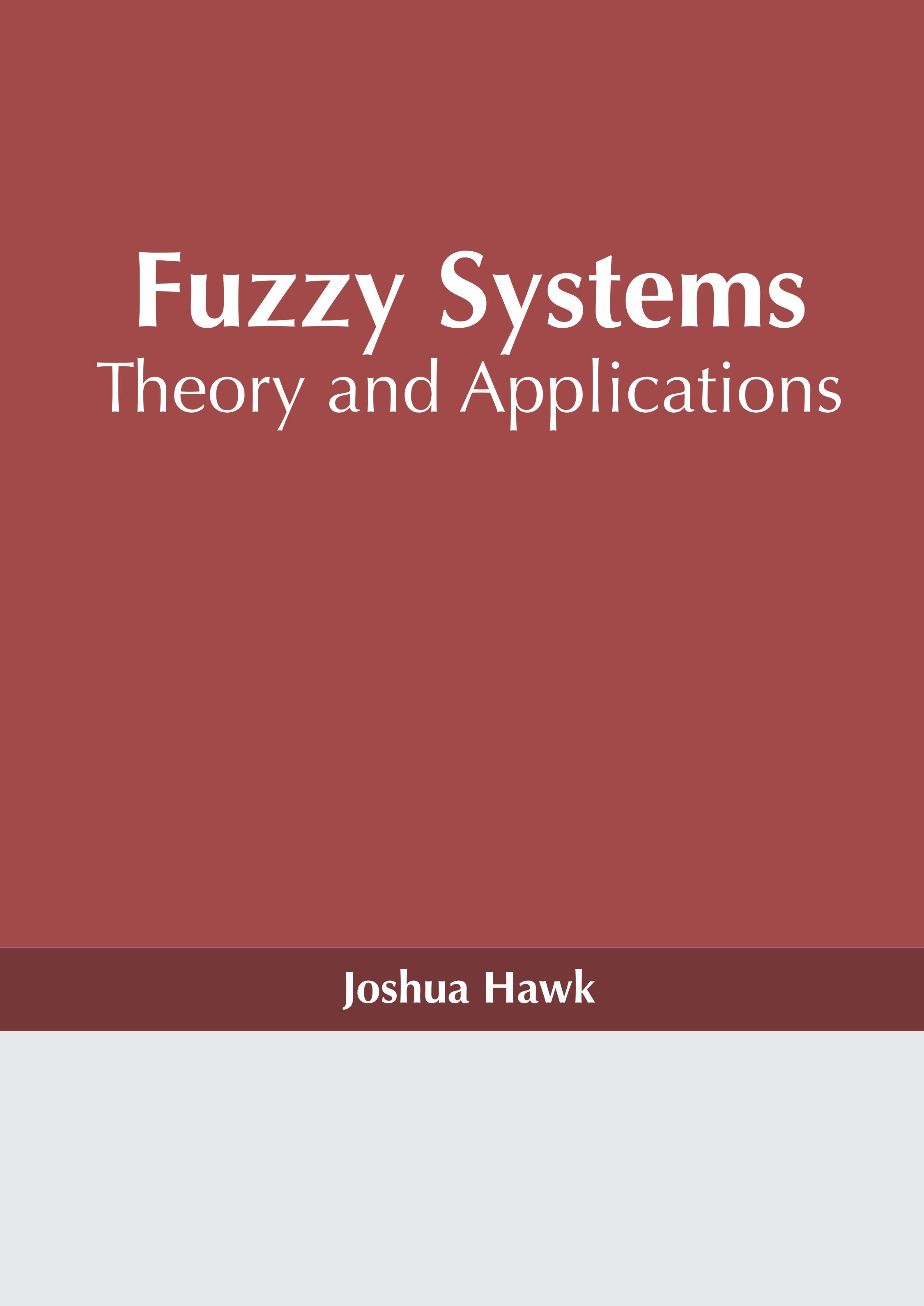Fuzzy Systems: Theory and Applications Author : Joshua Hawk Subject : Fuzzy Logic A control system which operates on fuzzy logic is known as a fuzzy system or a fuzzy control system. Fuzzy logic is a mathematical system which does not operate on absolute binary values of 0 or 1, but Read More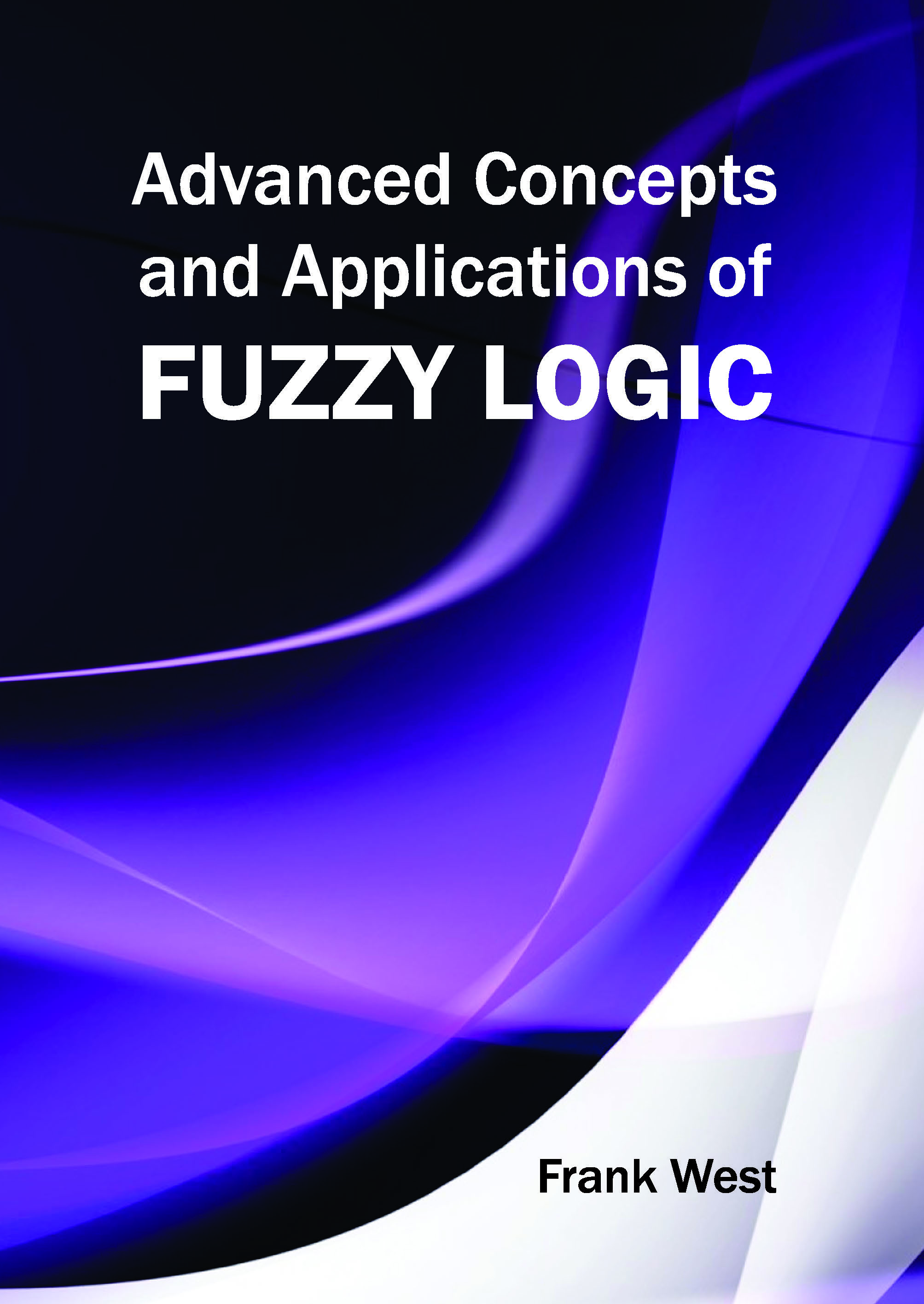Advanced Concepts and Applications of Fuzzy Logic Author : Frank West Subject : Fuzzy Logic ISBN :9781632380104 The advanced concepts and applications of fuzzy logic are elucidated in this comprehensive book. This book presents novel perceptions and ideas of Fuzzy Logic Control for their implementation in growth Read More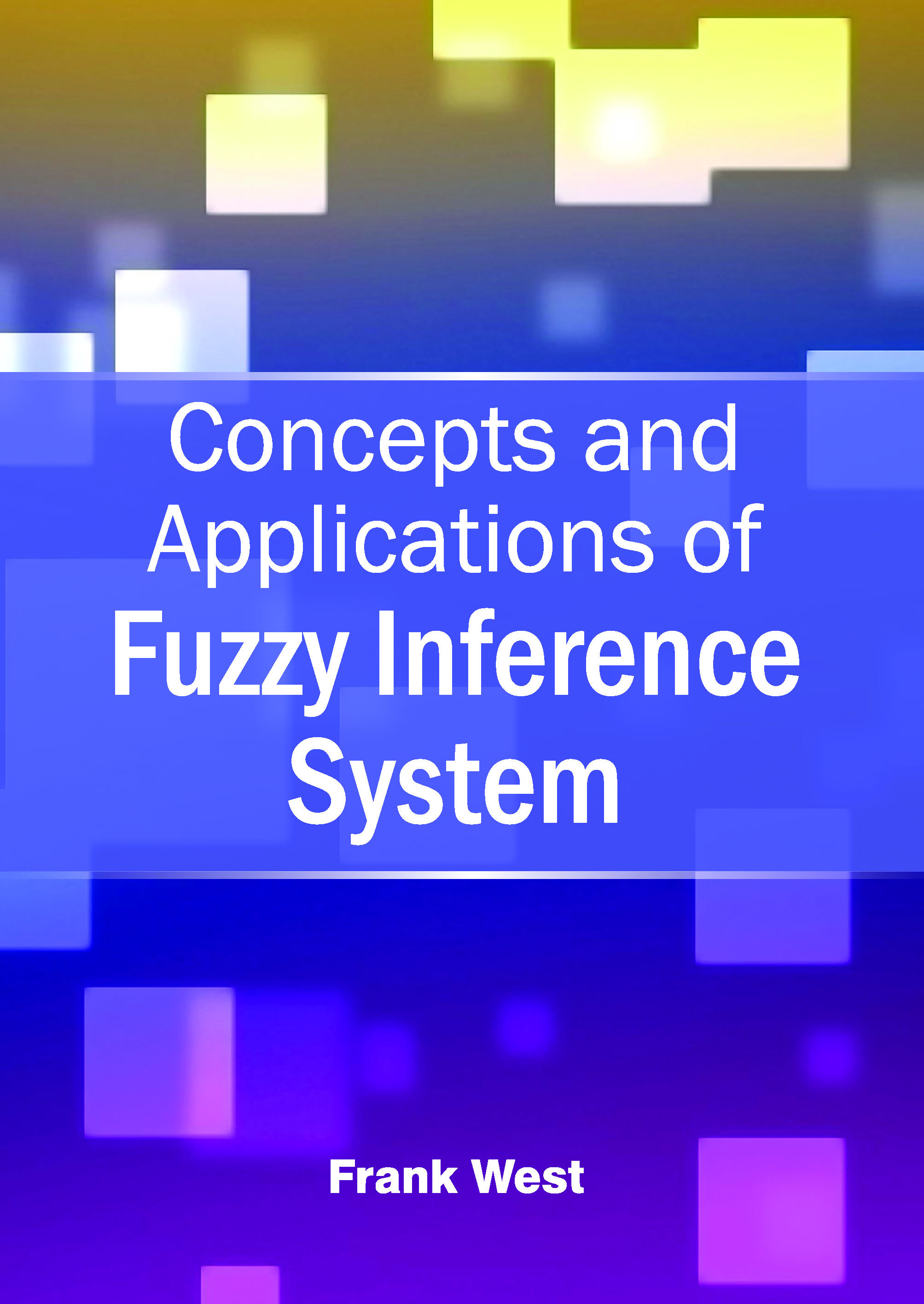Concepts and Applications of Fuzzy Inference System Author : Frank West Subject : Fuzzy Logic ISBN :9781632380920 This book aims to compile all important researches on the versatile and wide subject of engineering and management using Fuzzy Inference System (FIS). The book talks about the theoretical approaches of Read More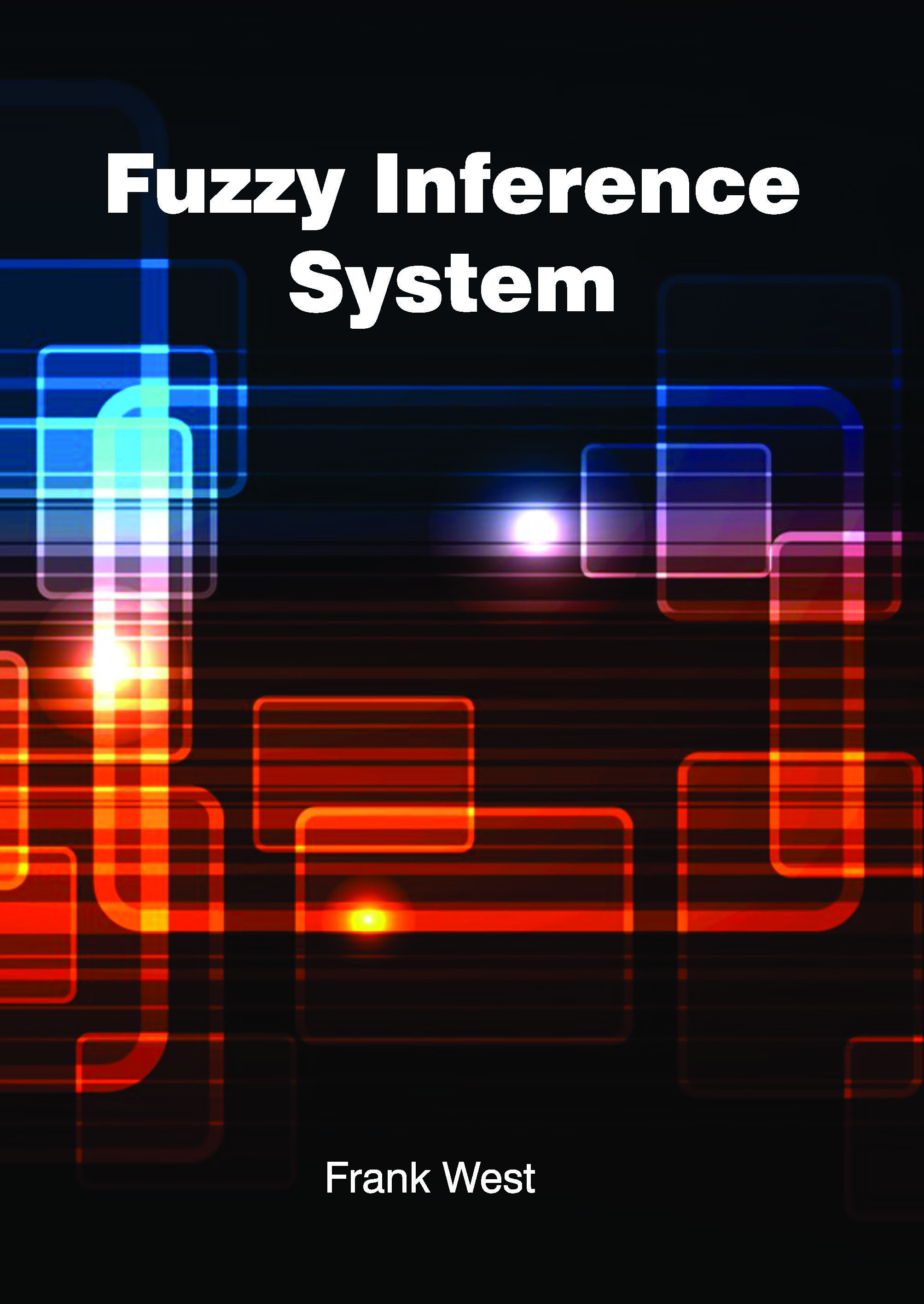Fuzzy Inference System Author : Frank West Subject : Fuzzy Logic ISBN :9781632382108 The book compiles all important researches on the versatile and wide subject of engineering and management using Fuzzy Inference System (FIS). The book talks about the theoretical approaches of FIS, deals Read More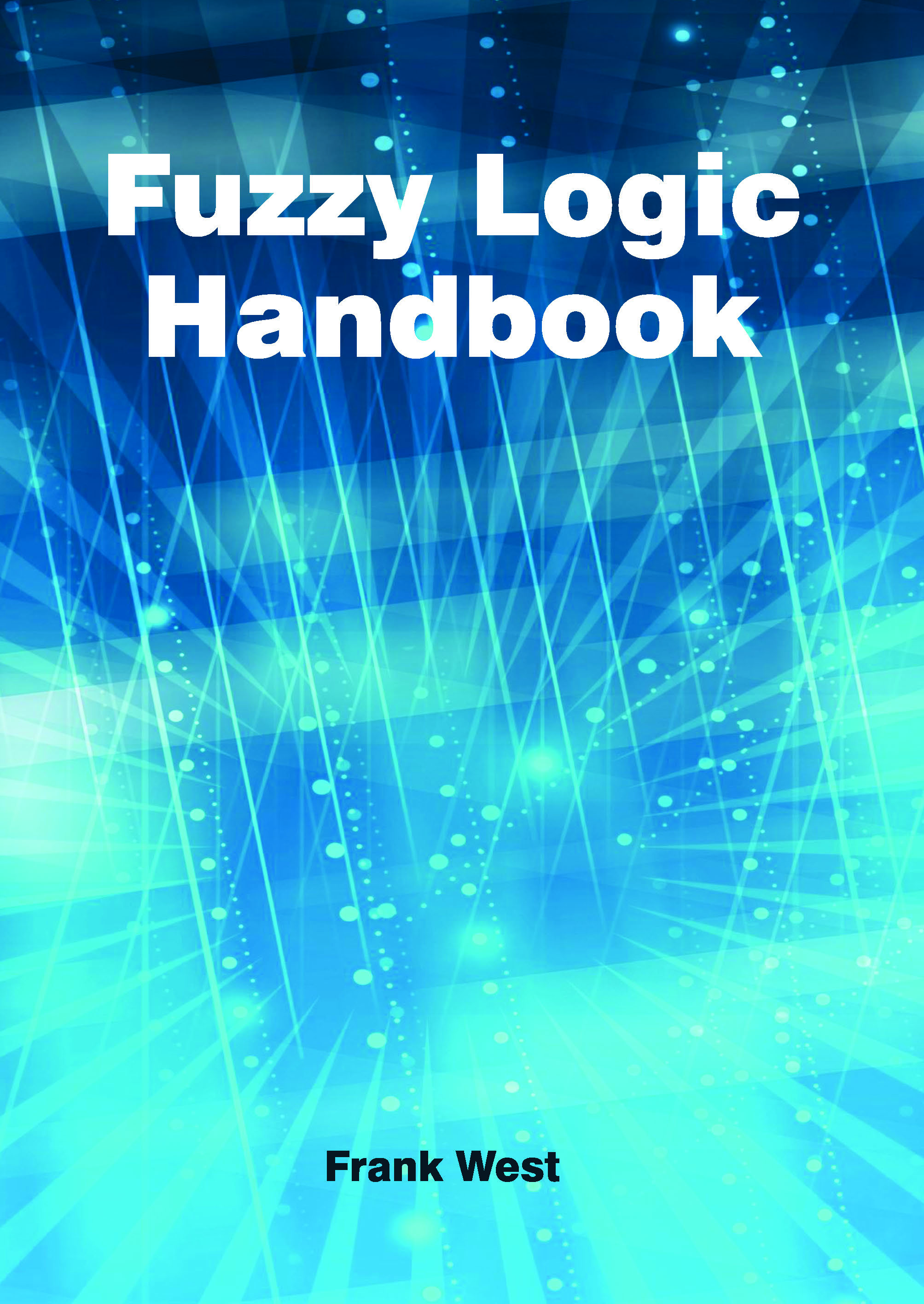Fuzzy Logic Handbook Author : Frank West Subject : Fuzzy Logic ISBN :9781632382115 Fuzzy logic has developed into an important means of solving problems in all domains. It has a huge impact on the design of autonomous intelligent systems. This book discusses hybrid algorithms, techniques, Read More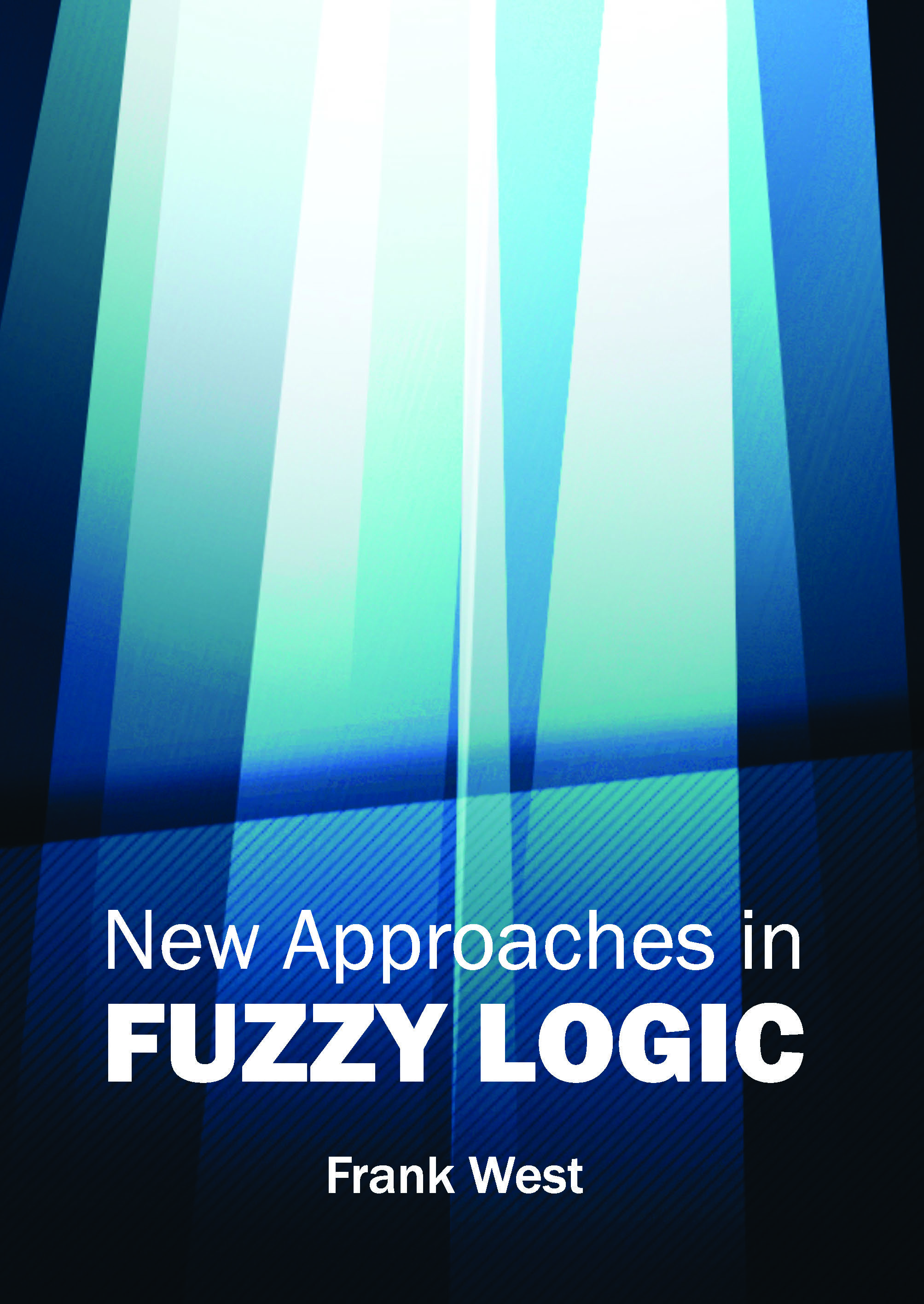New Approaches in Fuzzy Logic Author : Frank West Subject : Fuzzy Logic ISBN :9781632383426 Fuzzy logic has significant applications in numerous fields. The novel approaches in fuzzy logic have been described in this insightful book. The effectiveness of fuzzy logic in the advancement of rising Read MoreFuzzy Systems: Theory and Applications Author : Joshua Hawk Subject : Fuzzy Logic A control system which operates on fuzzy logic is known as a fuzzy system or a fuzzy control system. Fuzzy logic is a mathematical system which does not operate on absolute binary values of 0 or 1, but Read More
Results 1 - 7 of 7